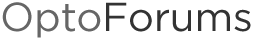# Sort table Subroutine

//This code takes an input table and the number of elements in that table and populates an output table that contains the input table values in sorted orde
for i = 0 to NumberOfElements - 1 step 1 //Number of elements is 1 based for an intuitive entry in the passed parameter. Subtract 1 for the zero based table
//initialie all counters
l = 0; //Lower than
s = 0; //Same as

for j = 0 to NumberOfElements - 1 step 1
if (unsorted_Table [i] > unsorted_table [j]) then
l= l+1; //count the Number of array elemts less than the cunrrent element of intrest
//This indicates the position of the sorted array that this number will occupy.

elseif(unsorted_Table [i] == unsorted_table [j]) then
s = s+1; //Count the Number of array elemts that are the same as the cunrrent element of intrest
// This gives the number of times that the sorted array will repeat the number
endif

next
k = l; //Set the position in the sorted array that the current number will occupy

//Note: this (repeat loop) section can be removed if only unique values are desired in the output table.
repeat //populate the sorted array at the proper position with consideration to the number of times tat a number is repeated.
Sorted_Table[k] = unsorted_Table [i];
IncrementVariable(k);

until (k == (l + s));
next